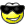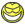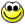# angle of the dangle

#### sotc

##### Dormant hero!!
can some one help me figure the formula to find the height of a tree with this info? the 90* is the only known angle, distance to trunk and distance to top are only known distances

#### Stumper

##### Treehouser
Pythagoran theory=the square of the hypotheneus is equal to the sum of the other sides.

B

##### Guest
Pythagoran theory=the square of the hypotheneus is equal to the sum of the other sides.
What he says.

You using a rangefinder to get the distance to the top?

#### lumberjack

##### Young man on the go
What's the distances you have?

#### sotc

##### Dormant hero!!
same thing
<object width="425" height="355"><param name="movie" value="http://www.youtube.com/v/0HYHG3fuzvk&hl=en"></param><param name="wmode" value="transparent"></param><embed src="http://www.youtube.com/v/0HYHG3fuzvk&hl=en" type="application/x-shockwave-flash" wmode="transparent" width="425" height="355"></embed></object>

#### lumberjack

##### Young man on the go
I have to watch the vid to get the two distances you have?

#### sotc

##### Dormant hero!!
say 30 yards to the trunk and 45 yards to the top

#### Stumper

##### Treehouser
Willie, If you have 2 sides and a 90 degree you can always back calculate the 3rd.-Think it through.

#### lumberjack

##### Young man on the go
A^2+B^2=C^2

45 is the hypotenuse, or C. 30 yards is a leg and would be either A or B.

A^2= 30x30= 900
C^2=45x45= 2025

So, we have A and C, so let's get B.

30^2+ B^2=45^2
900 + B^2=2025
B^2=2025-900
B^2=1125
B= Square Root of 1125
B= 33.54yards

Thus the tree is 33.54 yards tall.

#### sotc

##### Dormant hero!!
thanks carl, might take me a bit to get proficient at it but ill keep that formula in the truck for a while#### lumberjack

##### Young man on the go
What would be the height if you were 39.5' from the tree and the top of the tree was 49.5'?

#### sotc

##### Dormant hero!!
punk, i was starting to calculate that#### sotc

##### Dormant hero!!
did you just change that? id have swore you said 39.5 and 39.5
give me a sec to do it.

#### lumberjack

##### Young man on the go
Don't do 39.5 and 39.5, you'll wind up with a triangle that doesn't have a 90* angle (tree would be leaning)and I doubt your ready for the trig.

Plus, I'd have to really think about getting that right. Thus I changed one 39 to 49.

29.83?

#### sotc

##### Dormant hero!!
i used a square root calculater online, is there an easy way to find square root?

#### sotc

##### Dormant hero!!
Don't do 39.5 and 39.5, you'll wind up with a triangle that doesn't have a 90* angle (tree would be leaning)and I doubt your ready for the trig.

Plus, I'd have to really think about getting that right. Thus I changed one 39 to 49.
i thought that would be 39.5 as C would be a 45 degree angle?

#### lumberjack

##### Young man on the go
Right you are thenWhat about if you know the tree is 45' tall, and you're 45' away from it, how far is it from you to the top of the tree via line of sight?

#### lumberjack

##### Young man on the go
I use the one on my computer. There isn't an easy way for most folks to do square roots in their head.

The 39.5x39.5 triangle would be something aweful, I'll figure it out while you figure the last problem I gave ya.

The angles in a triangle equal 180, fwiw.

63.63

#### lumberjack

##### Young man on the go
You're getting it, I'm still working on the 39.5x39.5.